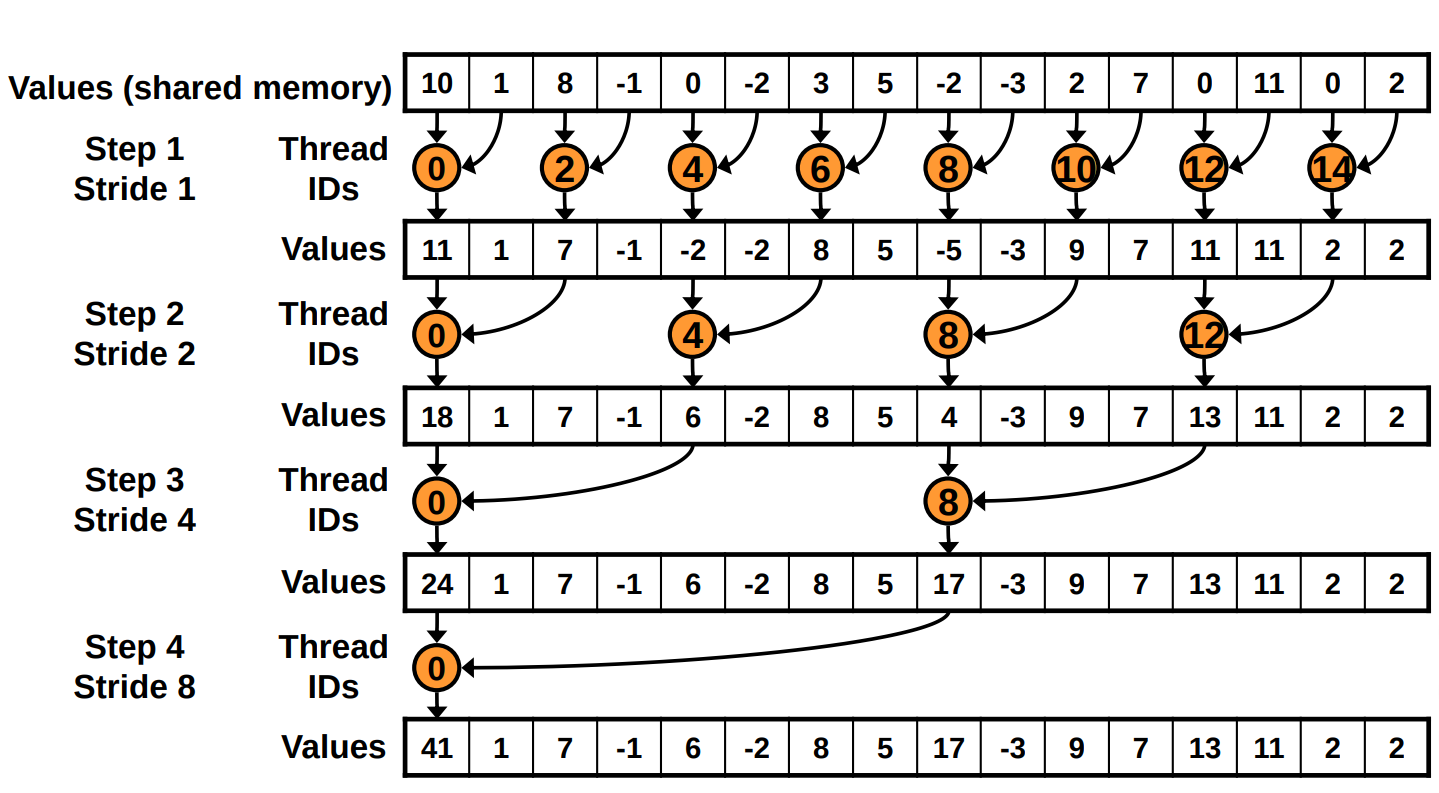# Parallel reduction

## Exercice - parallel reduction

In this exercice a parallel reduction kernel will be implemented. Write a kernel performing the sum operation.

Starting point:

#include <stdio.h>

void cpu_sum(int *x, int n)
{
int result = 0;
for(unsigned int i=0; i < n; ++i) {
result += x[i];
}
printf("CPU Sum is %d \n", result);
}

int main()
{
int h[] = {10, 1, 8, -1, 0, -2, 3, 5, -2, -3, 2, 7, 0, 11, 0, 2};

int size = sizeof(h);
int count = size/sizeof(int);

int* d;
cudaMalloc(&d, size);
cudaMemcpy(d, h, size, cudaMemcpyHostToDevice);

sum <<<1, count >>>(d);

int result;
cudaMemcpy(&result, d, sizeof(int), cudaMemcpyDeviceToHost);
cout << "GPU Sum is " << result << endl;

//cpu_sum(h, count);
cudaFree(d);
return 0;
}